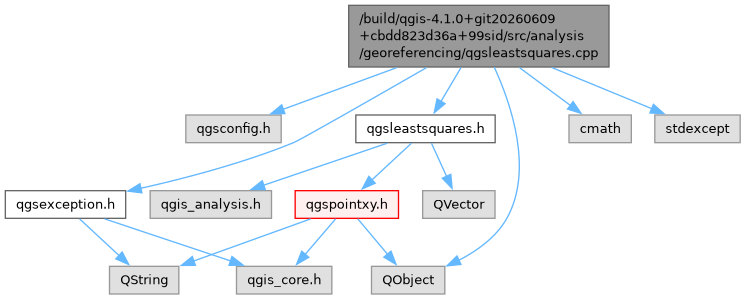QGIS API Documentation 3.35.0-Master (6cf940c20d6)
Searching...
No Matches
qgsleastsquares.cpp File Reference
`#include "qgsleastsquares.h"`
`#include "qgsconfig.h"`
`#include "qgsexception.h"`
`#include <QObject>`
`#include <cmath>`
`#include <stdexcept>`
Include dependency graph for qgsleastsquares.cpp:Go to the source code of this file.

## Functions

void normalizeCoordinates (const QVector< QgsPointXY > &coords, QVector< QgsPointXY > &normalizedCoords, double normalizeMatrix, double denormalizeMatrix)
Scales the given coordinates so that the center of gravity is at the origin and the mean distance to the origin is sqrt(2).

## ◆ normalizeCoordinates()

 void normalizeCoordinates ( const QVector< QgsPointXY > & coords, QVector< QgsPointXY > & normalizedCoords, double normalizeMatrix, double denormalizeMatrix )

Scales the given coordinates so that the center of gravity is at the origin and the mean distance to the origin is sqrt(2).

Also returns 3x3 homogeneous matrices which can be used to normalize and de-normalize coordinates.

Definition at line 205 of file qgsleastsquares.cpp.